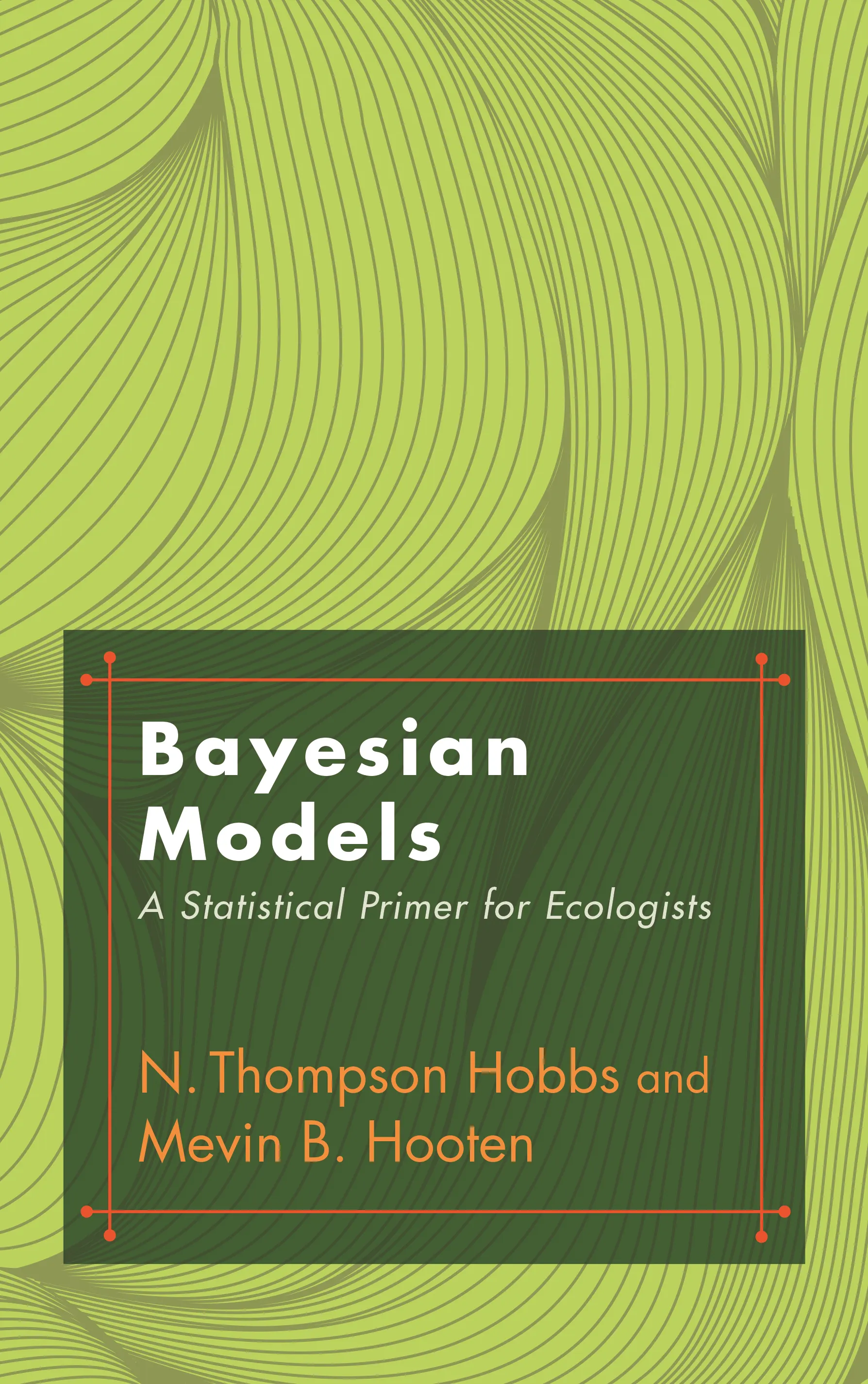#### Bayesian Models

N. Thompson Hobbs

50% off with code MAY50

# Bayesian Models: A Statistical Primer for Ecologists## Hardcover

50% off with code MAY50

Sale Price:
\$26.00/£22.50
Price:
\$52.00/£45.00
ISBN:
Published:
Aug 4, 2015
2015
Pages:
320
Size:
6 x 9.25 in.
Illus:
44 line illus. 5 tables.

Bayesian modeling has become an indispensable tool for ecological research because it is uniquely suited to deal with complexity in a statistically coherent way. This textbook provides a comprehensive and accessible introduction to the latest Bayesian methods—in language ecologists can understand. Unlike other books on the subject, this one emphasizes the principles behind the computations, giving ecologists a big-picture understanding of how to implement this powerful statistical approach.

Bayesian Models is an essential primer for non-statisticians. It begins with a definition of probability and develops a step-by-step sequence of connected ideas, including basic distribution theory, network diagrams, hierarchical models, Markov chain Monte Carlo, and inference from single and multiple models. This unique book places less emphasis on computer coding, favoring instead a concise presentation of the mathematical statistics needed to understand how and why Bayesian analysis works. It also explains how to write out properly formulated hierarchical Bayesian models and use them in computing, research papers, and proposals.

This primer enables ecologists to understand the statistical principles behind Bayesian modeling and apply them to research, teaching, policy, and management.

• Presents the mathematical and statistical foundations of Bayesian modeling in language accessible to non-statisticians
• Covers basic distribution theory, network diagrams, hierarchical models, Markov chain Monte Carlo, and more
• Deemphasizes computer coding in favor of basic principles
• Explains how to write out properly factored statistical expressions representing Bayesian models